# Symmetry Worksheets

#### What is Symmetry?

Any object that you can divide into two pieces will consider it asymmetry of that object. It helps transform things or shapes like reflection or rotation. Using symmetry for geometrical shapes helps us identifying them correctly. Symmetry also expresses the side, area, and volume, etc. There are two major aspects of geometry, similarity, and congruence. When two objects are the same with changed sizes is called similarity, when the size and shape of the two figures are the same, so we call that a congruence. We can make a coordinate plane by intersecting two number lines at a right angle. There are two number lines on the x and y-axis that are vertical and horizontal. The coordinate (0,0) is the intersection of two axes. Connect the dots on a coordinate plane to make shapes. It means we can plot lines, dots, and points with the help of a coordinate plane. We can solve all geometric problems by using theorems and formulas. Theorems are statements as proof of using previously famous facts. In the case of a right-angle triangle, Pythagoras Theorem gives proof that a2 + b2 = c2. There the variables a and b are right-angle triangle's side, and c is its hypotenuse.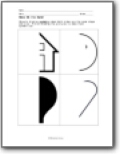###### Make Me the Same!

Objects display symmetry when both sides are the same shape and size. Finish drawing the pictures to make them symmetrical.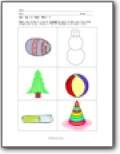###### Cut me in Two! Part 1

When you draw a line of symmetry both sides are the same shape and size. Draw a line of symmetry on each item.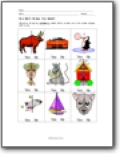###### Are Both Sides the Same?

Objects display symmetry when both sides are the same shape and size.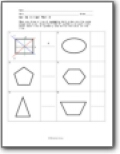###### Cut me in two! Part 2

When you draw a line of symmetry both sides are the same shape and size. Draw the lines of symmetry on each item. Count each line of symmetry and write the total on the line.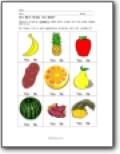###### Are Both Sides the Same?

Objects display symmetry when both sides are the same shape and size. Do these fruits and vegetables display vertical symmetry?